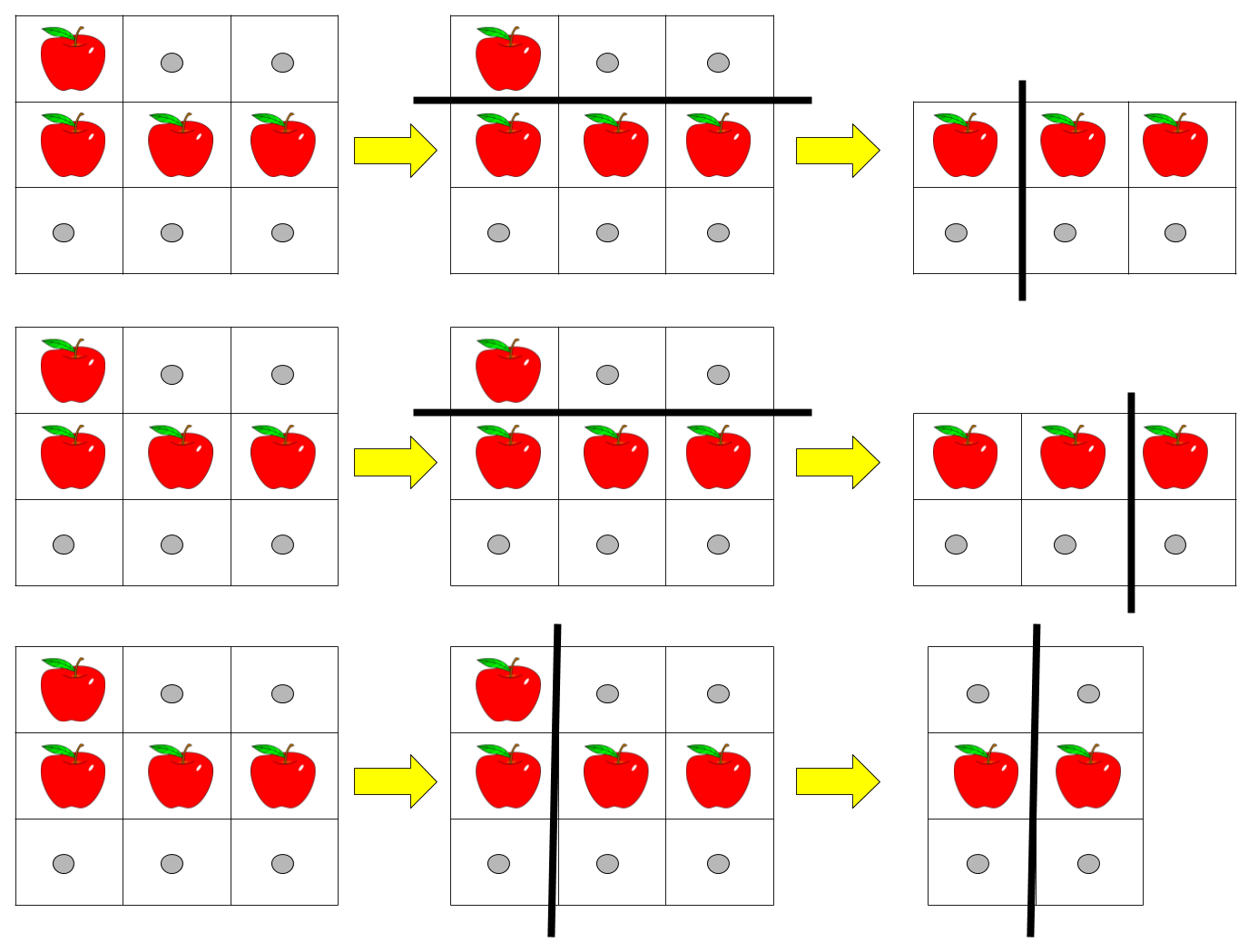# 切披萨的方案数

## 1444. 切披萨的方案数 (Hard)```输入：pizza = ["A..","AAA","..."], k = 3

```

```输入：pizza = ["A..","AA.","..."], k = 3

```

```输入：pizza = ["A..","A..","..."], k = 1

```

• `1 <= rows, cols <= 50`
• `rows == pizza.length`
• `cols == pizza[i].length`
• `1 <= k <= 10`
• `pizza` 只包含字符 `'A'` 和 `'.'` 。

[动态规划]